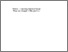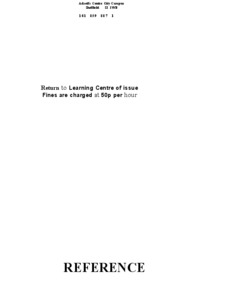# The polyhedral Gauss map and discrete curvature measures in geometric modelling.

ECHEVERRIA, Gilberto. (2007). The polyhedral Gauss map and discrete curvature measures in geometric modelling. Doctoral, Sheffield Hallam University (United Kingdom)..Preview
PDF (Version of Record)
10694479.pdf - Accepted Version

Related URLs:

## Abstract

The Work in this thesis is concentrated on the study of discrete curvature as an important geometric property of objects, useful in describing their shape. The main focus is on the study of the methods to measure the discrete curvature on polyhedral surfaces. The curvatures associated with a polyhedral surface are concentrated around its vertices and along its edges. An existing method to evaluate the curvature at a vertex is the Angle Deficit, which also characterises vertices into flat, convex or saddle. In discrete surfaces other kinds of vertices are possible which this method cannot identify. The concept of Total Absolute Curvature (TAC) has been established to overcome this limitation, as a measure of curvature independent of the orientation of local geometry. However no correct implementation of the TAC exists for polyhedral surfaces, besides very simple cases.For two-dimensional discrete surfaces in space, represented as polygonal meshes, the TAC is measured by means of the Polyhedral Gauss Map (PGM) of vertices. This is a representation of the curvature of a vertex as an area on the surface of a sphere. Positive and negative components of the curvature of a vertex are distinguished as spherical polygons on the PGM. Core contributions of this thesis are the methods to identify these polygons and give a sign to them. The PGM provides a correct characterisation of vertices of any type, from basic convex and saddle types to complex mixed vertices, which have both positive and negative curvature in them.Another contribution is a visualisation program developed to show the PGM using 3D computer graphics. This program helps in the understanding and analysis of the results provided by the numerical computations of curvature. It also provides interactive tools to show the detailed information about the curvature of vertices.Finally a polygon simplification application is used to compare the curvature measures provided by the Angle Deficit and PGM methods. Various sample meshes are decimated using both methods and the simplified results compared with the original meshes. These experiments show how the TAC can be used to more effectively preserve the shape of an object. Several other applications that benefit in a similar way with the use of the TAC as a curvature measure are also proposed.

Item Type: Thesis (Doctoral) Thesis (Ph.D.)--Sheffield Hallam University (United Kingdom), 2007. Sheffield Hallam Doctoral Theses EPrints Services 10 Apr 2018 17:19 26 Apr 2021 11:46 https://shura.shu.ac.uk/id/eprint/19598View Item